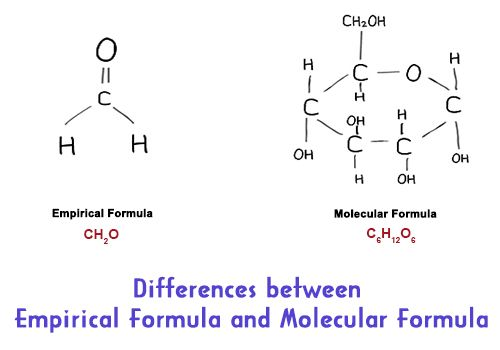# Differences between Empirical Formula and Molecular Formula

Molecular formulas tell you how many atoms of each constituent are in a compound, and empirical formulas tell you the simplest or most summary ratio of elements in a compound. The molecular formula gives us the actual number of different atoms in a molecule. And, the empirical formula is exact when describing ionic compounds, which cannot be broken into a single molecule unit.

Differences between Empirical Formula and Molecular Formula:

Empirical Formula – It is the simplest formula for a compound which is defined as the ratio of subscripts of the smallest possible whole number of the elements present in the formula.

1. Empirical Formula shows the simplest ratio of elements in a molecular compound. The empirical formula of a chemical compound is a representation of the simplest whole number ratio between the elements comprising the compound.
2. The formula which gives the simple whole number ratio of the atom of various elements present in one molecule of the compound is called empirical formula.
3. The empirical formula expresses the elements by which it is formed.
4.  It is determined by knowing the percentage of the elements of the compounds.
5. Sometimes the molecular formula and empirical formula of any compound become identical. E.g. HCI
6. The empirical formula of different compounds can be the same, e.g., Empirical formula of Benzene and ethyne is CH.
7. Empirical formula is only for compound.
8. The formula which gives the simple whole number ratio of the atom of various elements present in one molecule of the compound is called empirical formula.
9. The empirical formula expresses the elements by which it is formed. It is determined by knowing the percentage of the elements of the compounds.
10. The process of Derivation: The empirical formula is derived first from the weight percentages of the elements present in the compound.

Uses: The empirical formula is not often used in reaction schemes.Molecular Formula – It is the formula derived from molecules and is representative of the total number of individual atoms present in a molecule of a compound.

1. Molecular Formula shows us the number and type of atoms that make up a molecule. The molecular formula is the representation of the actual whole number ratio between the elements of the compound.
2. The formula which gives the actual number of atoms of various elements present in one molecule of the compound is called molecular formula.
3. This formula expresses the molecular structure of the compound or element.
4. It can be determined by knowing the percentage of elements and the molecular mass of the compounds.
5. The molecular formula of any compound sometimes becomes identical with empirical formula and sometimes it becomes multiple of the empirical formula. e.g., H2O (empirical formula H20) and C6H6 (empirical formula CH).
6. The molecular formula of any compound is always fixed except isomerism. e.g., The molecular formula of Benzene and ethyne are respectively C6H6 and C2H2.
7. The molecular formula is for both compounds and elements.
8. The formula which gives the actual number of atoms of various elements present in one molecule of the compound is called molecular formula.
9. This formula expresses the molecular structure of the compound or element. It can be determined by knowing the percentage of elements and the molecular mass of the compounds.
10. The process of Derivation: The molecular formula is related to the total weight of the compound in question and often is derived after obtaining the empirical formula.

Uses: The molecular formula is commonly used in reactions and other chemical recordings.